[图形学笔记系列] 实现一个简单的软光栅渲染效果-03

实践部分

图元装配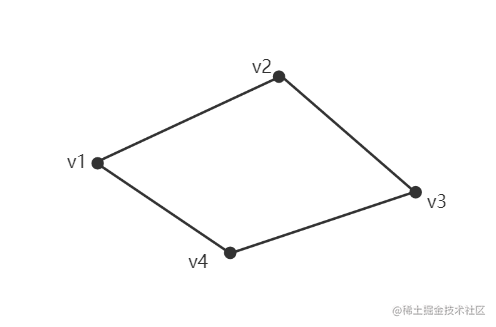以三角面为基本图元进行图元装配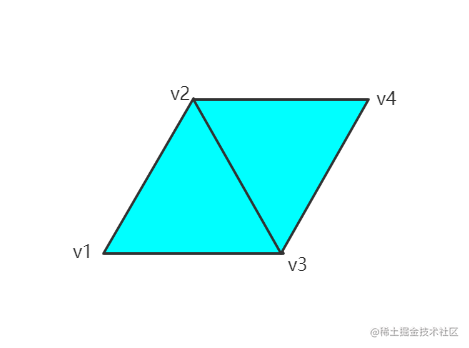光栅化

深度测试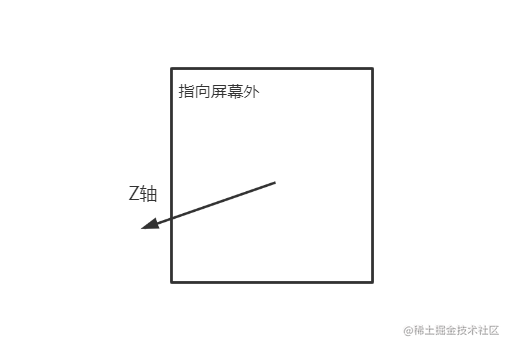片元着色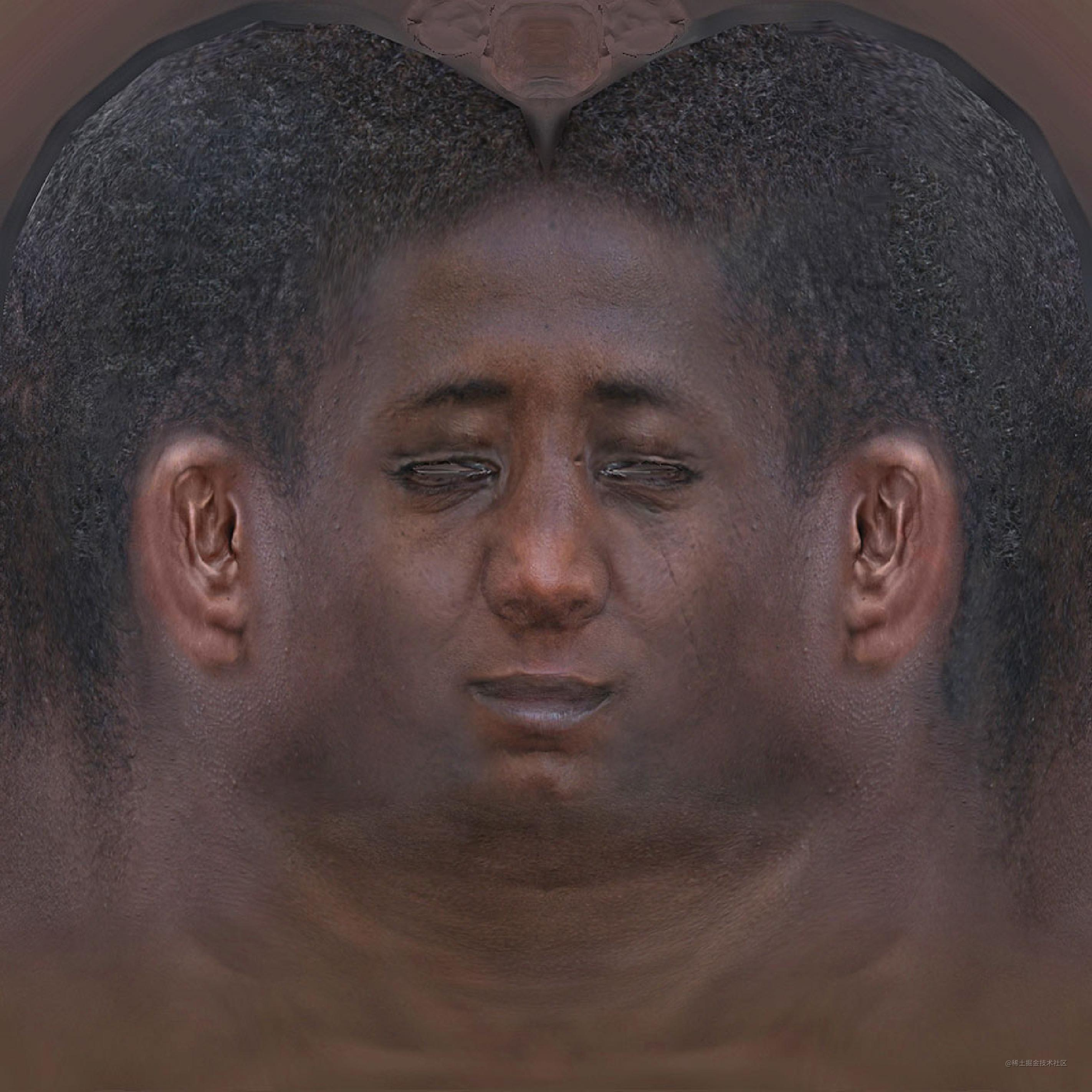但我觉得最好从二维矩阵的角度来理解它，纹理映射我推荐这一篇《纹理映射》。在光栅的过程中，我们会得到三角面的所有片元，每个片元需要的值都要依赖顶点直接或者间件的插值获得，比如用于纹理映射的UV坐标，需要依据每个顶点对应的UV坐标，通过权值插值得到片元的UV坐标，再去获取相应纹理贴图对应坐标下的值。这个插值的过程也有坑就是你插值的权值的问题，我用的是利用重心法求出的权值来进行插值，但这是一种没有进行透视校正的插值方法，贴图会出现透视不正确的现象，关于透视校正插值我推荐这一篇文章《图形学 - 关于透视矫正插值那些事》。我这里也没有进行透视校正，主要更多的是体会思想，渲染管线的很多流程步骤都可以展开成很深的领域，学海无涯啊。获取纹理贴图对应坐标下的值，是一个采样的问题，这里面也是一个深坑，涉及到数字信号处理的一些理论。

这一部分的代码

function barycentric(A, B, C, P) {
let s = []
for(let i = 0; i < 2; i++) {
let tmp = glMatrix.vec3.create()
tmp = C[i]-A[i]
tmp = B[i]-A[i]
tmp = A[i]-P[i]
s.push(tmp)
}

let u = glMatrix.vec3.create()
glMatrix.vec3.cross(u, s, s)

if ( Math.floor(Math.abs(u)) != 0 ) {
let result = glMatrix.vec3.fromValues(1-(u+u)/u, u/u, u/u)
return result
}

return glMatrix.vec3.fromValues(-1, -1, -1)

}

function triangle_raster(pts, uvs, normals, light_dir, zBuffer, width, getTexture, fragementShader, drawPixel) {
let bboxmin = [Infinity, Infinity]
let bboxmax = [-Infinity, -Infinity]
for(let i = 0; i < 3; i++) {               //找到三角形面的边框
for(let j = 0; j < 2; j++) {
bboxmin[j] = Math.min(bboxmin[j], pts[i][j])
bboxmax[j] = Math.max(bboxmax[j], pts[i][j])
}
}

let p = glMatrix.vec2.create()

for (p = Math.floor(bboxmin); p <= bboxmax; p++) {
for(p = Math.floor(bboxmin); p <= bboxmax; p++) {
let c = barycentric(pts, pts, pts, p) //由重心坐标得到的权值

let z = pts*c + pts*c + pts*c  //插值出P的深度值

if (c < 0 || c < 0 || c < 0 || zBuffer[Math.floor(p) + Math.floor(p)*width] > z)
continue;

zBuffer[Math.floor(p) + Math.floor(p)* width] = z;

let uv = glMatrix.vec3.create()           //插值出P的uv坐标
glMatrix.vec3.transformMat3(uv, c, uvs)

let normal = glMatrix.vec3.create()       //插值出P的法线向量
glMatrix.vec3.normalize(normal, normal)
glMatrix.vec3.transformMat3(normal, c, normals)

glMatrix.vec3.normalize(light_dir, light_dir)
drawPixel(p, p, fragementShader(uv, getTexture, normal, light_dir))
}
}
}

let color = texture(uv)
let intensity = glMatrix.vec3.dot(normal, light_dir)
glMatrix.vec3.scale(color, color, intensity)
return color
}

结果展示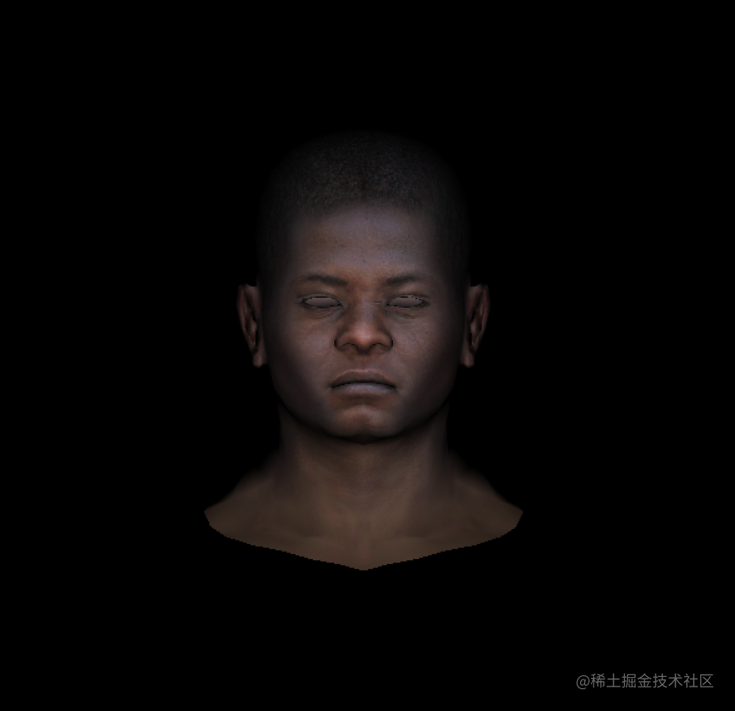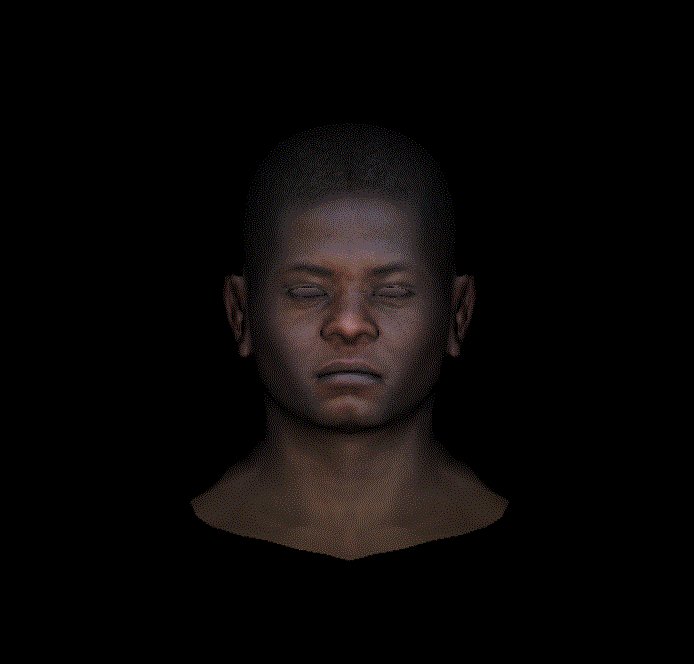实现一个简单的软光栅渲染效果的源码和数据

github.com/yangla2zhen…

参考资料

GAMES101-现代计算机图形学入门-闫令琪

Fundamentals of Computer Graphics, Fourth Edition

Tiny renderer or how OpenGL works: software rendering in 500 lines of code